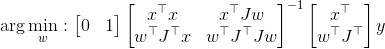# Optimization involving the inverse of an SDP matrix

Hi,
I want to optimize the following expression with respect to a vector ‘w’ involving the inverse of an SDP matrix.(the matrix to be inverted is SDP)
‘x’ and ‘y’ are nx1, ‘w’ is mx1 and ‘J’ is nxm.
There seems not to be any direct way of optimizing an inverse with CVX and the proposed solutions in related posts do not apply directly to my problem. Any hint on how to formulate this problem in a way that can be solved with CVX is highly welcome.
Thanks!

`matrix_frac` and use of Scuir Complements can deal with some problems involving inverses. I don;t see how to use either for this problem.

Unless you prove otherwise, I will presume this problem is not convex, and therefore cannot be entered or solved in CVX. Why isn't CVX accepting my model? READ THIS FIRST!

This can be formulated and submitted for solution to a non-convex solver using YALMIP. In order to do so, you will need to introduce a matrix variable for the inverse, and then add a nonlinear equality constraint that the inverse matrix times the matrix equals the identity matrix. No guarantee that the solver will succeed. The better your starting value for the variables, the better chance the solver will succeed.

1 Like